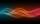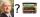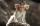# Sequence

In the arithmetic sequence is a1=-1, d=4. Which member is equal to the number 203?

Result

n =  52

#### Solution:Leave us a comment of example and its solution (i.e. if it is still somewhat unclear...):Be the first to comment!#### To solve this example are needed these knowledge from mathematics:

Do you have a linear equation or system of equations and looking for its solution? Or do you have quadratic equation?

## Next similar examples:

1. NumberCalculate the integer number which, divided by 34 gives 10 and the rest 25.
2. Unknown xIf we add to unknown number 21, then divide by 6 and then subtract 51, we get back an unknown number. What is this unknown number?
3. MistakeNicol mistake when calculate in school. Instead of add number 20 subtract it. What is the difference between the result and the right result?
4. Candy and boxesWe have some number of candy and empty boxes. When we put candies in boxes of ten, there will be 2 candies and 8 empty boxes left, when of eight, there will be 6 candies and 3 boxes left. How many candy and empty boxes left when we put candies in boxes of.
5. IntegerFind the integer whose distance on the numerical axis from number 1 is two times smaller as the distance from number 6.
6. Chickens and rabbitsIn the yard were chickens and rabbits. Together they had 54 heads and 118 legs. How many chickens and how many rabbits was in the yard?
7. LineStraight line passing through points A [-3; 22] and B [33; -2]. Determine the total number of points of the line which both coordinates are positive integers.
8. CZK coinsThaddeus and Jolana together have 15 CZK. Jolana has half of Thaddeus money. Nevertheless Jolana has 3 coins and Thaddeus 2 coins. Which coin has Thaddeus and Jolana (Help: CZK coins has values 1,2,5,10,20,50 CZK)?
9. 15 numberWhat number is smaller (greater) by 15 than its half?
10. ModuloFind x in modulo equation: 47x = 4 (mod 9) Hint - read as: what number 47x divided by 9 (modulo 9) give remainder 4 .
11. Mysterious numberThe magician thinks the number: "The mysterious number is first divided by minus five, dividing the result by three, multiplying the number by ten, and dividing the resulting number by minus four. This gives result 5. Can you reveal the mysterious number?
12. Four integersFnd four consecutive integers so that the product of the first two is 70 times smaller than the product of the next two.
13. Two numbers 6Fill two natural numbers a, b: 7 + blank- blank = 5
14. Ladislav in AmsterdamLadislav was reward and went to conference in Amsterdam. Conferense fee was € 3484. Calculate how many books Ladislav could buy at the price of 48 and 52 euros if he don't want fill whole home library and he can buy only 70 books?
15. RepairmanRepairman has vowed to do repair work at the plant for 25 days. However work had to be shortened, and therefore he took helper worker. Together they made all the corrections for the whole days. How long it would take work to helper worker?
16. Balance of accountTheo had a balance of -\$4 in his savings account. After making a deposit, he has \$25 in his account. What is the overall change to his account?
17. EquatiomSolve equation with negatives: X/(-5) + 2 = -9